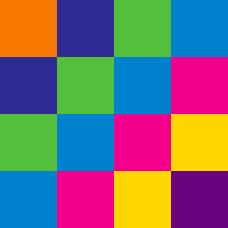Basic Mathematics

# Patterns Using Geometry Warmup

NOTE: For all problems in this quiz, all figures are to scale; sides that appear congruent are congruent, angles that appear to be right angles are right angles.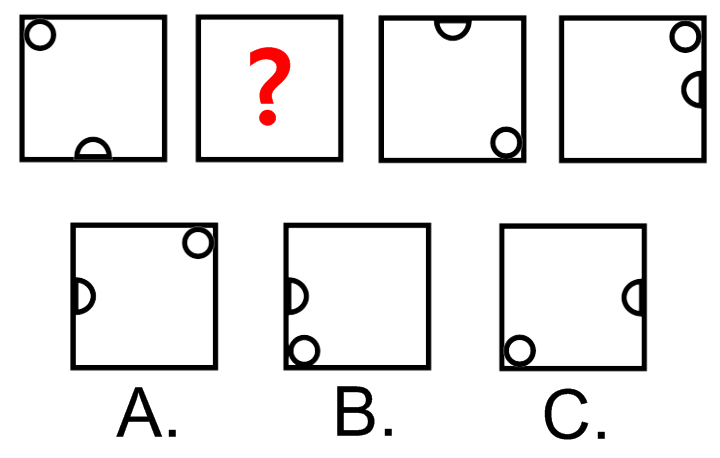The sequence shown repeats indefinitely.

Which choice goes in the question mark?

NOTE: For all problems in this quiz, all figures are to scale; sides that appear congruent are congruent, angles that appear to be right angles are right angles.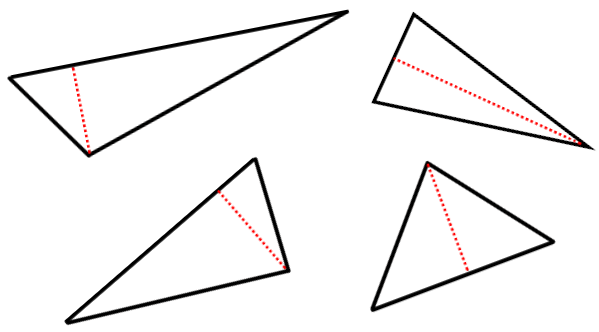Given the pattern above ...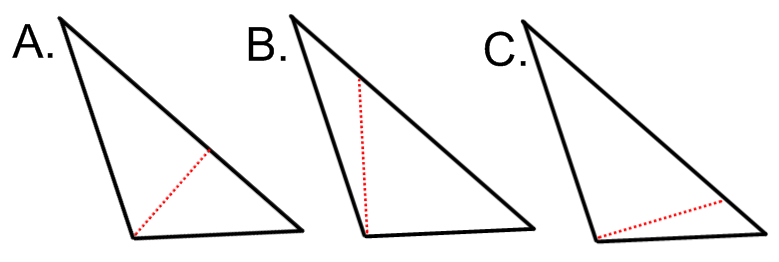... which of these choices fits best?

NOTE: For all problems in this quiz, all figures are to scale; sides that appear congruent are congruent, angles that appear to be right angles are right angles.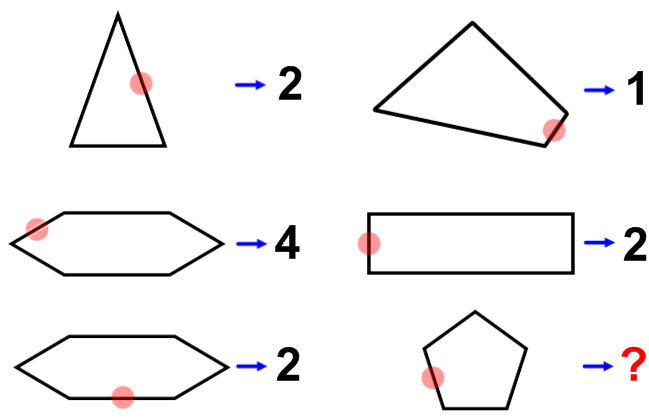The last figure is a regular pentagon. What goes at the question mark?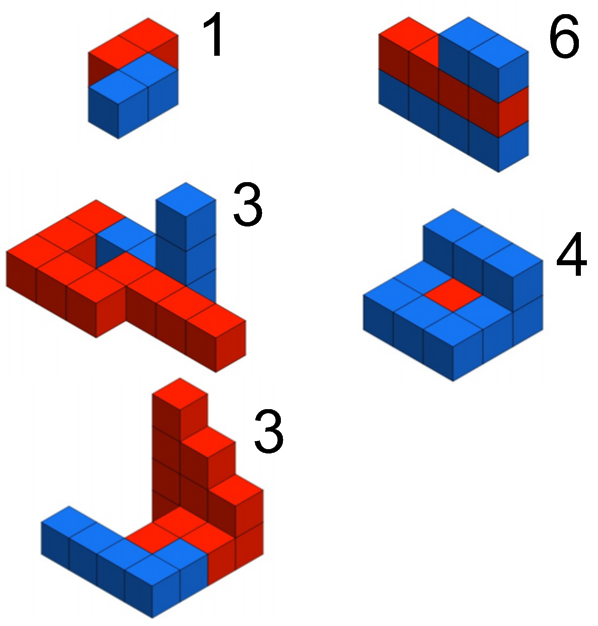Given the numbers corresponding to figures as shown above...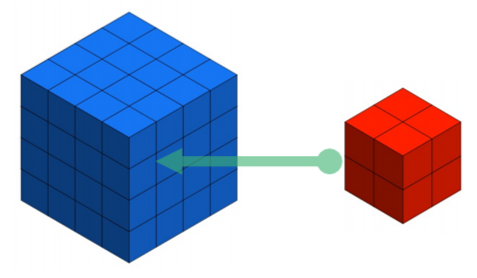What number corresponds to a 2x2 cube of red blocks surrounded by a 4x4 cube of blue blocks?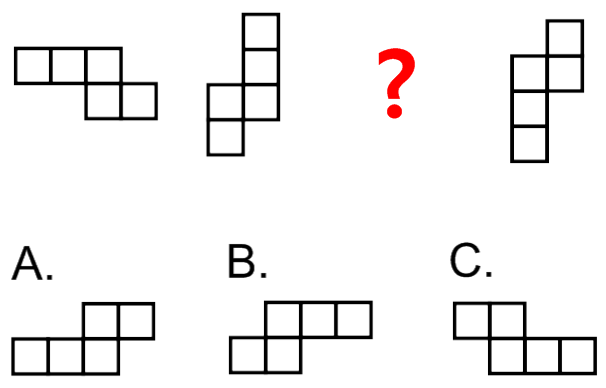What goes at the question mark?

×

Problem Loading...

Note Loading...

Set Loading...